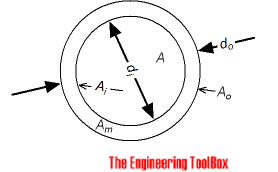Engineering ToolBox - Resources, Tools and Basic Information for Engineering and Design of Technical Applications!

# Pipe Equations

## Calculate cross-sectional areas, weight of empty pipes, weight of pipes filled with water, inside and outside surface areas.### Cross Sectional inside Pipe Area

Cross-sectional inside area of a pipe can be calculated as

Ai = π (di / 2)2

= π di 2 / 4                                 (1)

where

Ai = cross-sectional inside area of pipe (m2, in2)

di = inside diameter (m, in)

### Cross Sectional Pipe Wall Area

The cross-sectional wall area - or area of piping material - can be calculated as

Amπ (do / 2)2 - π (di / 2)2

= π (do 2 - di 2) / 4                               (2)

where

Am = cross-sectional wall area of pipe (m2, in2)

do = outside diameter (m, in)

### Weight of Empty Pipes

Weight of empty pipes per unit length can be calculated as

wpρm Am

= ρm (π (do / 2)2 - π (di / 2)2

= ρm  π (do2 - di2) / 4                              (3)

where

wp = weight of empty pipe per unit length (kg/m, lb/in)

ρs = density of pipe material (kg/m3, lb/in3)

### Weight of Liquid in Pipes

Weight of liquid in pipes per unit length can be calculated as

wl = ρl A

= ρl π (di / 2)2

= ρl π di2 / 4                                   (4)

where

wl = weight of liquid in pipe per unit length of pipe (kg, lb)

ρl = density of liquid (kg/m3, lb/in3)

### Weight of Pipe filled with Liquid

Weight of pipe filled with liquid per unit length can be calculated as

w = wl + wp                          (5)

where

w = weight of pipe and liquid per unit length of pipe (kg, lb)

### Outside Surface Area of Pipes

Outside surface area of steel pipes per unit length can be calculated as

Ao = 2 π (do / 2)

=  π do                            (6)

where

Ao = outside area of pipe - per unit length of pipe (m2, in2)

### Inside Surface Area of Pipes

Inside surface area of steel pipes per unit length can be calculated as

Ai2 π (di / 2)

=  π di                             (7)

where

Ai = inside area of pipe - per unit length of pipe (m2, in2)

## Related Topics

• ### Dimensions

Sizes and dimensions of pipes and tubes, and their fittings - inside and outside diameter, weight and more.

## Related Documents

• ### ANSI Schedule 40 Steel Pipes - Dimensions

Internal and external diameters, areas, weights, volumes and number of threads for ANSI schedule 40 steel pipes.
• ### Area Units Converter

Convert between units of area.
• ### Darcy-Weisbach Equation - Major Pressure and Head Loss due to Friction

The Darcy-Weisbach equation can be used to calculate the major pressure and head loss due to friction in ducts, pipes or tubes.
• ### Hazen-Williams Friction Loss Equation - calculating Head Loss in Water Pipes

Friction head loss (ftH2O per 100 ft pipe) in water pipes can be estimated with the empirical Hazen-Williams equation.

Ice coat weigth on horizontal pipe lines.
• ### Manning's Formula and Gravity Flow

Calculate cross-sectional average velocity flow in open channels.
• ### Pipe Formulas

Pipe and Tube Equations - moment of inertia, section modulus, traverse metal area, external pipe surface and traverse internal area - imperial units
• ### Pipe Weight Calculator

Weight calculating equation for steel pipes.
• ### Pipes - Fractional Equivalents

Fractional vs. decimal inches.
• ### Pipes - Nominal Wall Thickness

Nominal wall thickness of seamless and welded carbon and alloy steel pipes
• ### Pipes and Tubes - Water Content

Water content in steel pipes and copper tubes.
• ### Stainless Steel Pipes - Dimensions and Weights

Dimensions, wall thickness and weights of stainless steel pipes according to ASME B36.19 Stainless Steel Pipes.
• ### Welded Steel Pipes - Weights

Nominal weights of welded steel pipes ranging 26 - 60 inches.

## Engineering ToolBox - SketchUp Extension - Online 3D modeling!

Add standard and customized parametric components - like flange beams, lumbers, piping, stairs and more - to your Sketchup model with the Engineering ToolBox - SketchUp Extension - enabled for use with older versions of the amazing SketchUp Make and the newer "up to date" SketchUp Pro . Add the Engineering ToolBox extension to your SketchUp Make/Pro from the Extension Warehouse !

We don't collect information from our users. More about

## Citation

• The Engineering ToolBox (2005). Pipe Equations. [online] Available at: https://www.engineeringtoolbox.com/pipes-equations-d_873.html [Accessed Day Month Year].

Modify the access date according your visit.

10.2.10